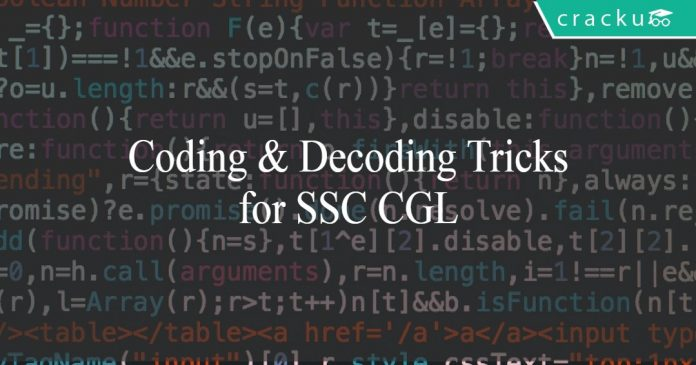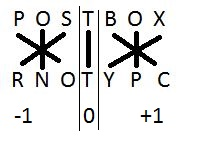# Coding decoding Tricks and Questions for SSC CGL

1
1363Coding Decoding Tricks & questions for SSC CGL

Every day, millions of critical information are floating around us in the air. To protect the privacy of the data, information is encrypted at the transmitting end and decrypted at the receiving end. Effectively what anyone who intercepts the message gets is gibberish, and some sense can be made out of it only if the key is available. Coding decoding for SSC CGL follows the same logic.

A word is encoded. Candidates are asked to encode another word using the logic used in the previous case. Cracking the logic early can reward aspirants handsomely in this topic. Candidates can prepare online for SSC CGL to score high in this section.

SSC repeats some questions from previous year papers of SSC CGL. Also, solving previous year papers acquaints the candidates with the exam pattern. Solving mock tests for SSC CGL will aid in the preparation process.

Let us take a look at the various types of commonly asked questions under coding decoding for SSC CGL in this post.

# Coding decoding for SSC CGL:

Most of the questions will be based on alphabetic series. Candidates must write down the alphabets and their corresponding positions to make things easy. We try to cover various questions asked in coding decoding for SSC CGL as much as possible.

### Question 1:

If CAB is coded as DBC, then how will be BAR coded?
We can see that every letter is replaced by the letter that follows it in the alphabetic series. Hence, BAR will be coded as CBS.

### Question 2:

If CAB is coded as DCE, then how will be BAR coded?
Here, the alphabet at position 1 is followed by the next alphabet, position 2 is followed by second next alphabet and so on. Hence, BAR will be coded as CCU.

### Question 3:

If PLAY is coded as QKBX, then RAIN is coded as ___________.
We can see that for odd positions, the alphabet is replaced by the succeeding alphabet and for even positions, the alphabet is replaced by preceding alphabet. Hence, RAIN will be coded as SZJM.

### Question 4:

If RAIN is coded as SZHM, then PRIVATE will be coded as __________.
Vowels are replaced by the letters preceding them and consonants are replaced by the letters succeeding them. So, PRIVATE will be coded as QSHWZUD.

### Question 5:

In a coding language, vowels are replaced by the letters that precede them in the alphabetical order and consonants are replaced by the letters that succeed them. Determine the word coded as QSHWZUD?

Here, most aspirants will choose PRIVATE as answer had choices been given. However, we must remember that we cannot be sure whether letter H has been used to code G or I. Similarly, we cannot determine for all the alphabets that precede a vowel. Hence, the correct answer cannot be determined.

### Question 6:

In a certain language, DESPERATE is coded as H J K I J O W L J. How will SEED be coded in the same language?

In this question, we cannot establish any relation between the position or the nature of the alphabet to the way it is coded. But we can see that E is replaced by J, S by K and D by H. Hence, the answer is K J J H.

Just as a food for thought, try to calculate the number of possibilities if each alphabet from A-Z is assigned to another unique alphabet.

### Question 7:

If POSTBOX is coded as RNOTYPC, then how will be PACIFIC coded?

This is a tricky one. SBI is known to ask such questions. The solution is given in the image below.Coding-decoding for SSC CGL

Using similar logic, PACIFIC will be coded as BZOIDJG.

### Question 8:

If BRIDGE is coded as B62, FOUNTAIN is coded as F84, then EDUCATION is coded as ___________.

From the 2 examples given, we can infer that the first digit represents the starting letter, the second digit represents the number of letters in the word and the third digit represents the number of vowels in the word. Hence, EDUCATION will be coded as E95.

## How to tackle set based questions in coding decoding for SSC CGL:

SSC asks set based questions. The first thing to do in such questions is to draft a table. As mind can remember only a limited number of items, it is essential that you have some point of reference. Otherwise, the data remembered while solving the first question cannot be recollected while solving the last one which results in solving the problem multiple times.

Let us have a look at an example:
In a certain language,
“ How are you “ is coded as “ uy li ka.”
“ I am fine” is coded as “ gi ru pa.”
“You are fine” is coded as “ ka li gi”.

How is “ How am I” coded in the language?
A)ru pa uy
B)uy pa li
C)gi ru ka
D)ka li pa

The trick is to start solving using common terms. Between sentences 1 and 3, “are you” is common. From this, we can infer that “how” is coded as “uy”. Similarly, between sentences 2 and 3, fine is common. From this, we can infer that “I am” is coded as “ru pa”. Hence, option A is the right answer.

To know about the other type of questions, aspirants can read Reasoning for SSC CGL.